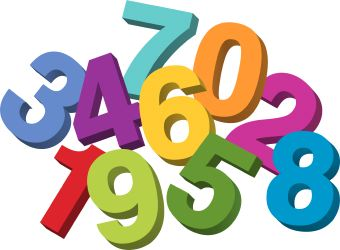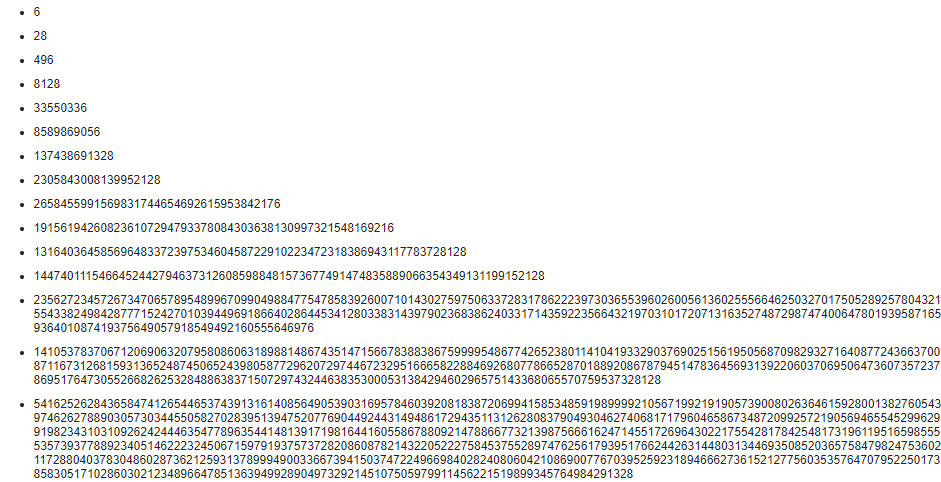# Find out about Perfect NumbersThe Definition of a Perfect Number:

In number theory, a perfect number is a positive integer that is equal to the sum of its proper positive divisors, that is, the sum of its positive divisors excluding the number itself. This is also known as its aliquot sum.## List of Perfect numbers